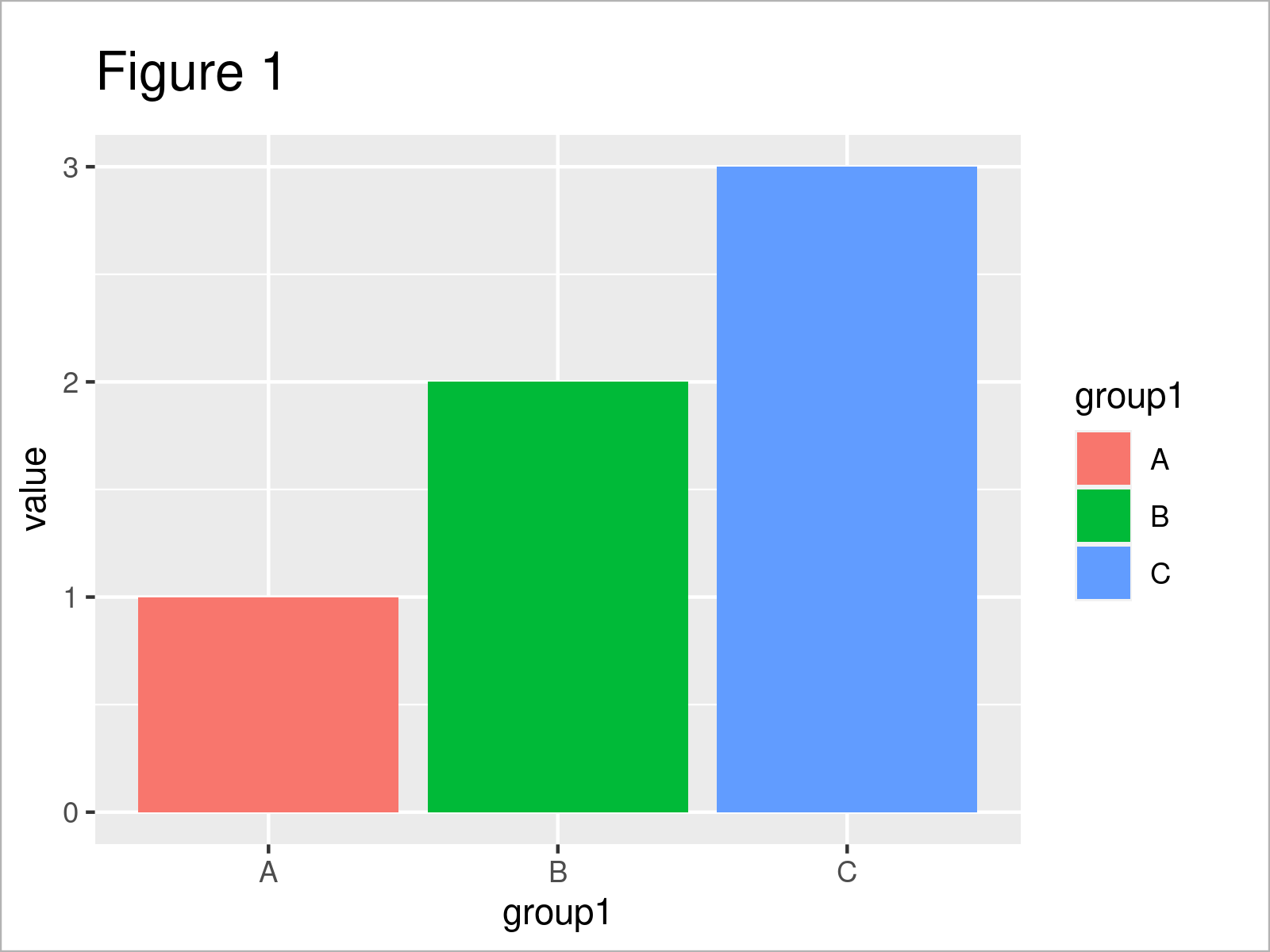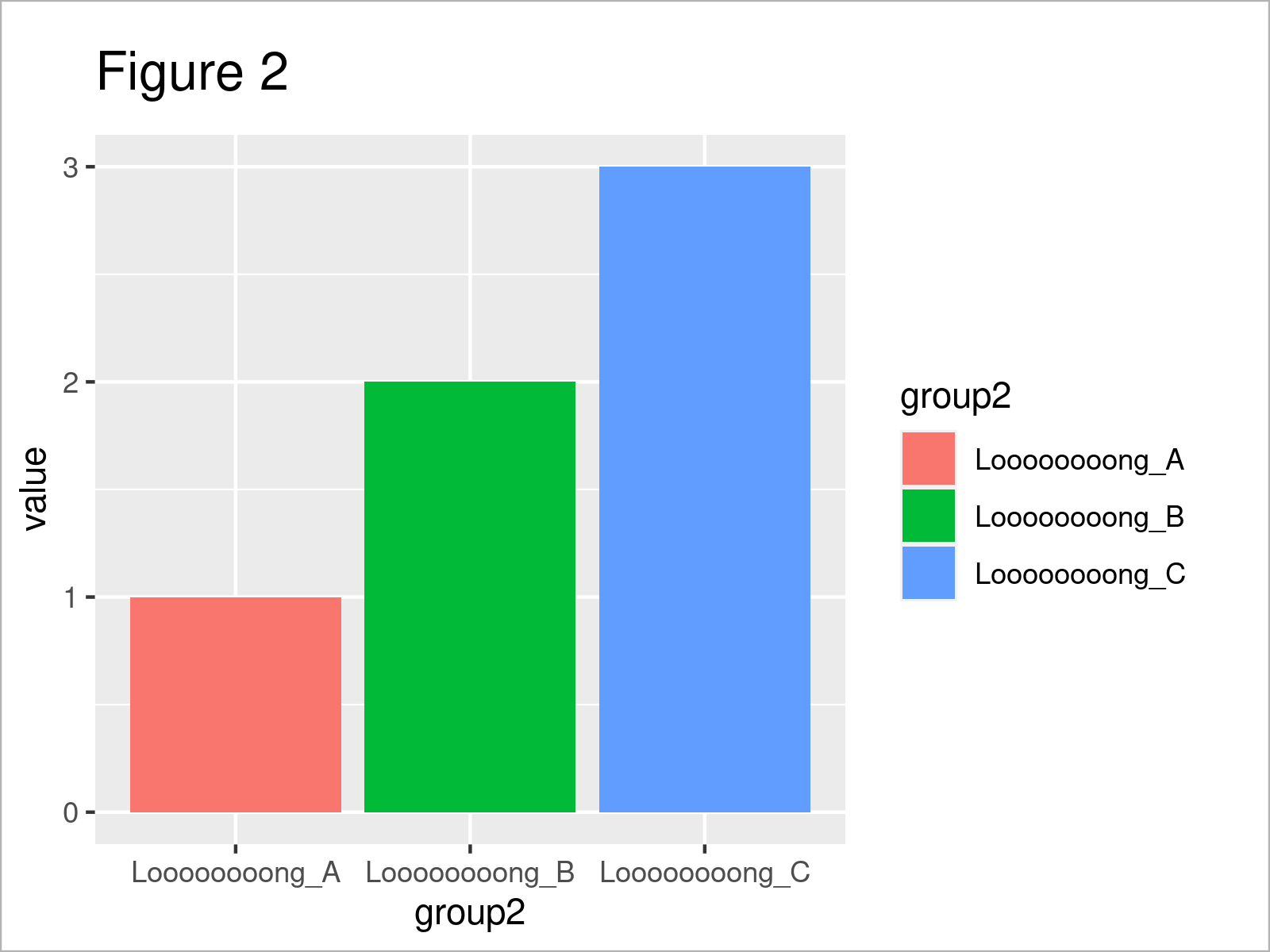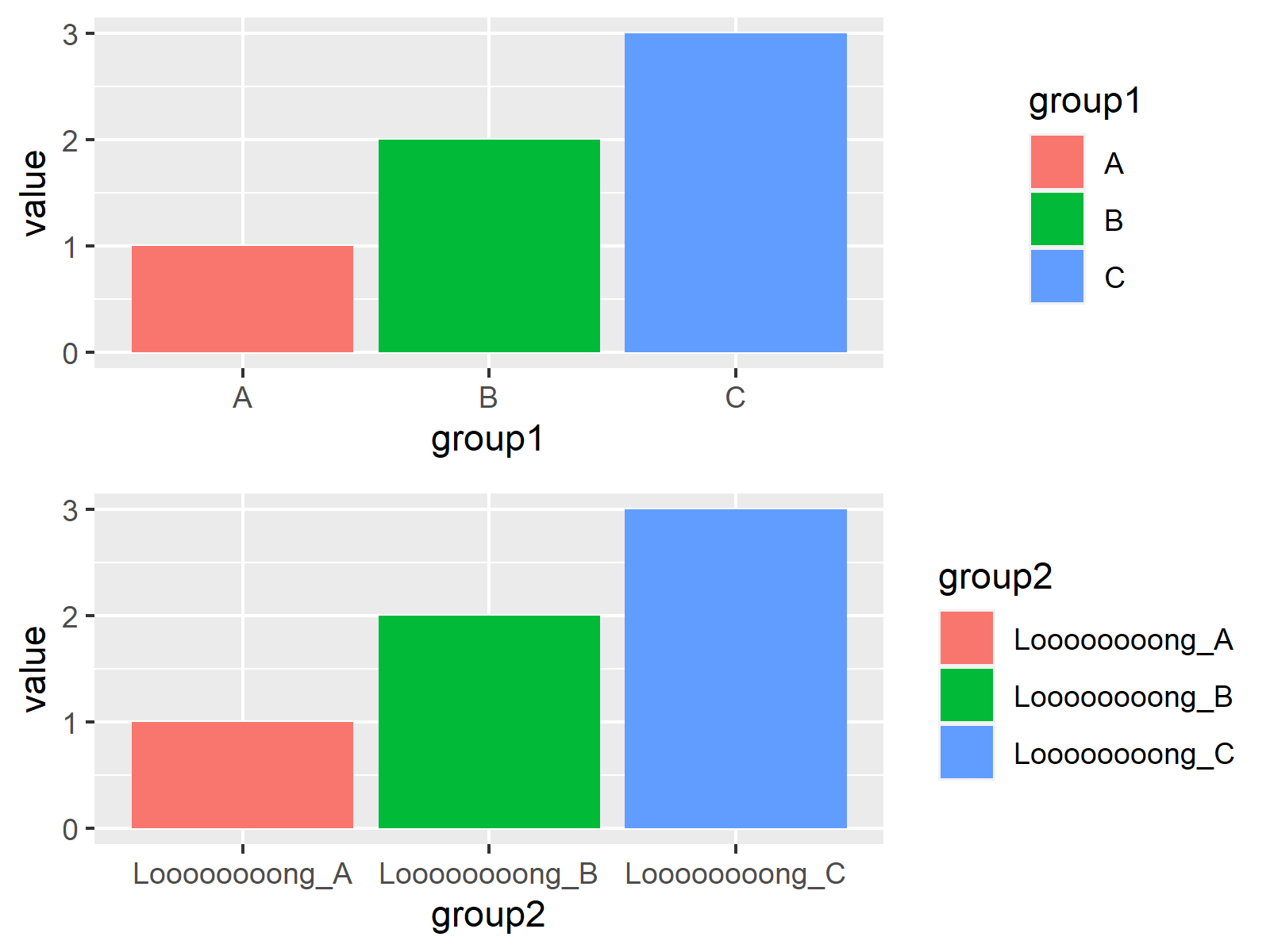# Draw Multiple ggplot2 Plots with Consistent Width in R (Example)

In this tutorial, I’ll demonstrate how to create a plot composition of multiple ggplot2 graphics with the same width in R programming.

Let’s take a look at some R codes in action.

## Exemplifying Data, Software Packages & Basic Graph

I’ll use the following data as basement for this tutorial:

```data <- data.frame(value = 1:3,    # Create example data
group1 = LETTERS[1:3],
group2 = c("Loooooooong_A",
"Loooooooong_B",
"Loooooooong_C"))
data                               # Print example data```Have a look at the table that got returned after running the previous R syntax. It shows that our example data consists of three rows and three columns with the names “value”, “group1”, and “group2”.

We also have to install and load the ggplot2 package, if we want to use the corresponding commands and functions:

```install.packages("ggplot2")        # Install & load ggplot2
library("ggplot2")```

Now, we can draw our data in two plots with different legends as shown below:

```ggp1 <- ggplot(data,               # Create first plot
aes(x = group1,
y = value,
fill = group1)) +
geom_bar(stat = "identity")
ggp1``````ggp2 <- ggplot(data,               # Create second plot
aes(x = group2,
y = value,
fill = group2)) +
geom_bar(stat = "identity")
ggp2```By executing the previous R programming code we have drawn Figures 1 and 2, i.e. two ggplot2 barplots. The two barcharts have a different width, since the two legends have a different width as well.

The following example illustrates how to harmonize the size of both graphs in a grid of plots.

## Example: Create Consistent-Width Plots using ggplot2 & cowplot Packages

In this example, I’ll show how to draw a layout of multiple graphics, where each graph has the same width.

First, we need to install and load the cowplot package.

```install.packages("cowplot")        # Install cowplot package

In the next step, we can use the plot_grid function of the cowplot package to draw a plot composition containing multiple ggplot2 plots side-by-side.

Note that we set the align argument to be equal to “v” to draw all graphs with a consistent width, no matter how large the legend is.

```plot_grid(ggp1, ggp2,              # Draw grid of plots
ncol = 1,
align = "v")```As shown in Figure 3, we have created a cowplot grid where all plot panels have the same size.

## Video & Further Resources

Do you need more explanations on the R syntax of this tutorial? Then I recommend having a look at the following video on my YouTube channel. In the video, I’m explaining the R programming codes of this page in a live session in RStudio.

Please accept YouTube cookies to play this video. By accepting you will be accessing content from YouTube, a service provided by an external third party.If you accept this notice, your choice will be saved and the page will refresh.

Furthermore, you may want to read the related articles on this website.

In this tutorial, I have explained how to draw a plot layout of multiple ggplot2 graphics with the same width in the R programming language. In case you have further questions and/or comments, don’t hesitate to let me know in the comments. In addition, please subscribe to my email newsletter for regular updates on the newest tutorials.

Subscribe to the Statistics Globe Newsletter